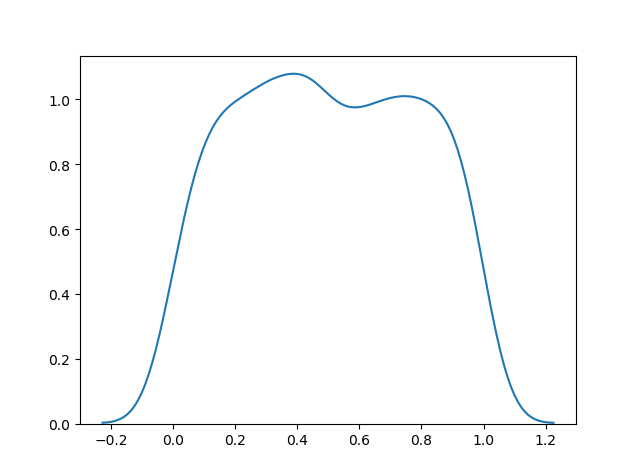THE WORLD'S LARGEST WEB DEVELOPER SITE

# Uniform Distribution

## Uniform Distribution

Used to describe probability where every event has equal chances of occuring.

E.g. Generation of random numbers.

It has three parameters:

`a` - lower bound - default 0 .0.

`b` - upper bound - default 1.0.

`size` - The shape of the returned array.

### Example

Create a 2x3 uniform distribution sample:

from numpy import random

x = random.uniform(size=(2, 3))

print(x)
Try it Yourself »

## Visualization of Uniform Distribution

### Example

from numpy import random
import matplotlib.pyplot as plt
import seaborn as sns

sns.distplot(random.uniform(size=1000), hist=False)

plt.show()

### ResultTry it Yourself »